# Depth of Field Calculator

Created by Jasmine J Mah and Kenneth Alambra
Reviewed by Wojciech Sas, PhD candidate and Adena Benn
Last updated: Jun 02, 2022

This depth of field calculator, or DoF calculator, will help you take more fantastic portrait and landscape shots by understanding your camera better when it comes to the depth of field. Take your depth of field photography to the next level with a solid understanding of:

• What depth of field is;
• What circle of confusion is;
• How to adjust the depth of field;
• The different depth of field formulas; and
• How to calculate the depth of field.

## What is the depth of field?

Depth of field is the distance between two planes, a closer one and a farther one, in which we can position objects to have an "acceptably sharp" image formed in a camera. Objects beyond the depth of field will appear blurred or out of focus. On a manual camera, we can set a wide or deep depth of field to capture more details of a scene, or we can have a narrow or shallow depth of field to focus on a particular object while blurring out the background or the foreground as shown in the image comparison below: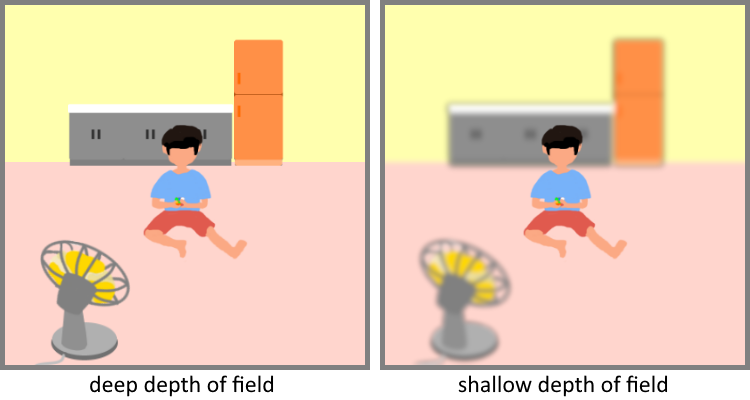We can achieve these depths of field by changing:

• Our camera's aperture area;
• The lens we use to explore different focal lengths; and
• Our distance to our subject or our focusing distance.

But first, how come we see blurred areas in the images we capture? We can explain that using the concept of the circle of confusion.

## What is circle of confusion?

Imagine an arbitrary point where we focus our camera. As light bounces off this point and travels towards our camera's aperture opening, it spreads out and starts to get blurry. The more it spreads out, the blurrier it gets. The maximum size spot of a circle this point can spread out before we consider it out of focus is called the circle of confusion.

The diameter of the circle of confusion, which we also call the circle of confusion limit, defines how deep the depth of field is. We can observe the circle of confusion in two instances: one between the camera and the focusing distance and one beyond the focusing distance. We call the distance from the camera toward the first circle of confusion the depth of field near limit, while the distance from the camera towards the second circle of confusion is the depth of field far limit, as shown in this illustration: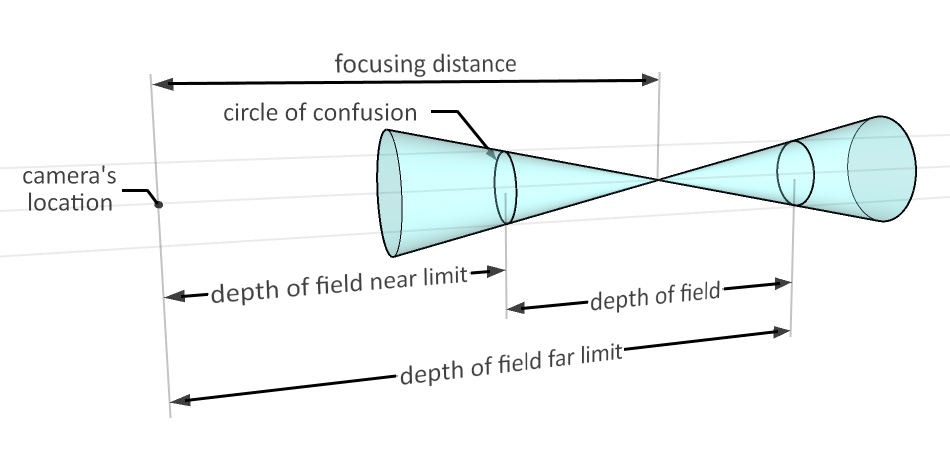The circle of confusion limit depends on various factors such as the camera's sensor size, the viewer's visual acuity, and the enlargement of the image produced by the camera to print size. We'll learn more about this in the following sections of this text.

## How to adjust depth of field?

#### Aperture area

Using a small aperture opening, we can achieve a deep depth of field where we can capture an acceptably sharp image of near and far objects, as illustrated below: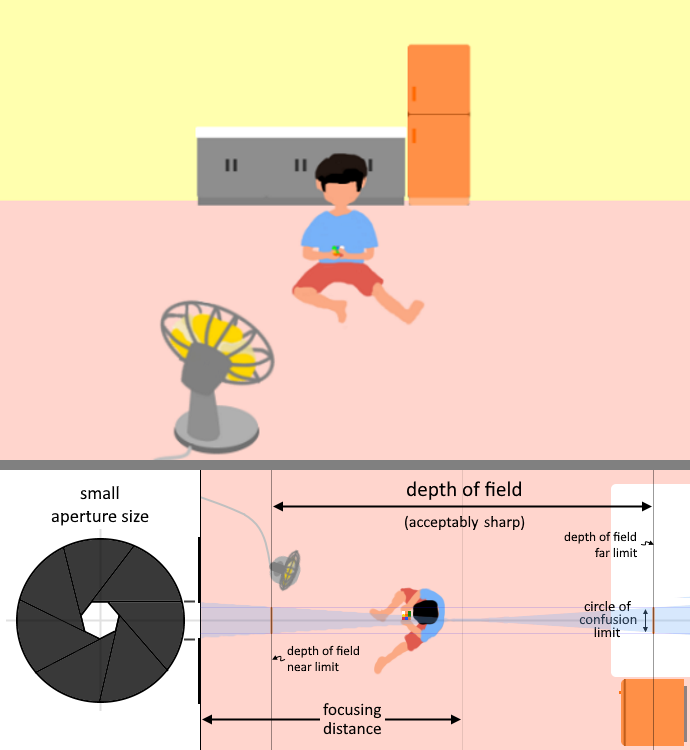#### Focusing distance

When taking pictures, we almost always want our subject to be within these two limits or the depth of field. We may also choose to keep our subject's foreground and background in focus or not.

From our previous example, if we shorten the focusing distance while maintaining the same aperture size, we decrease the depth of field, as shown below: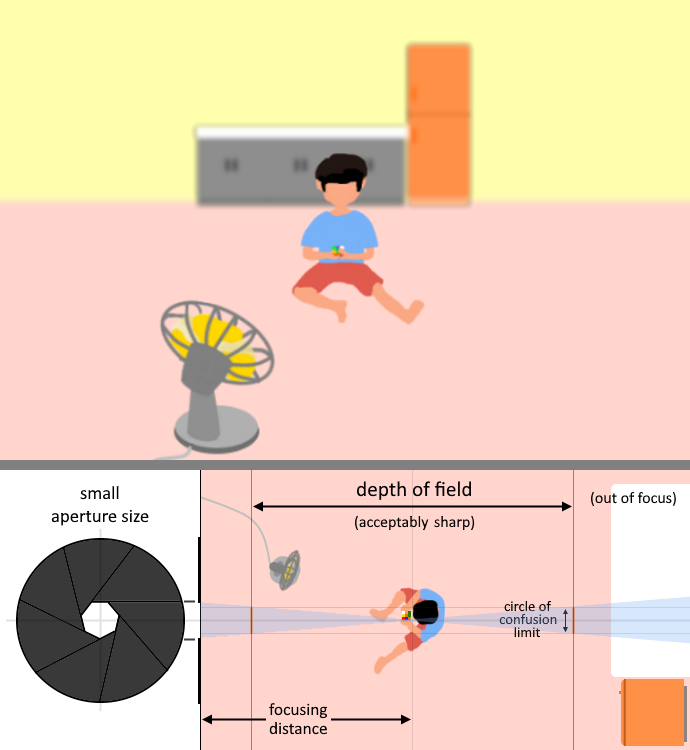We still have a deep depth of field; however, the kitchen countertop now lies beyond the depth of field and, therefore, out of focus. Now, let us increase the size of our aperture. Doing so allows light to spread wider, which results in a shorter distance to reach the circle of confusion limit, giving us a narrow depth of field, as we can see in the image below: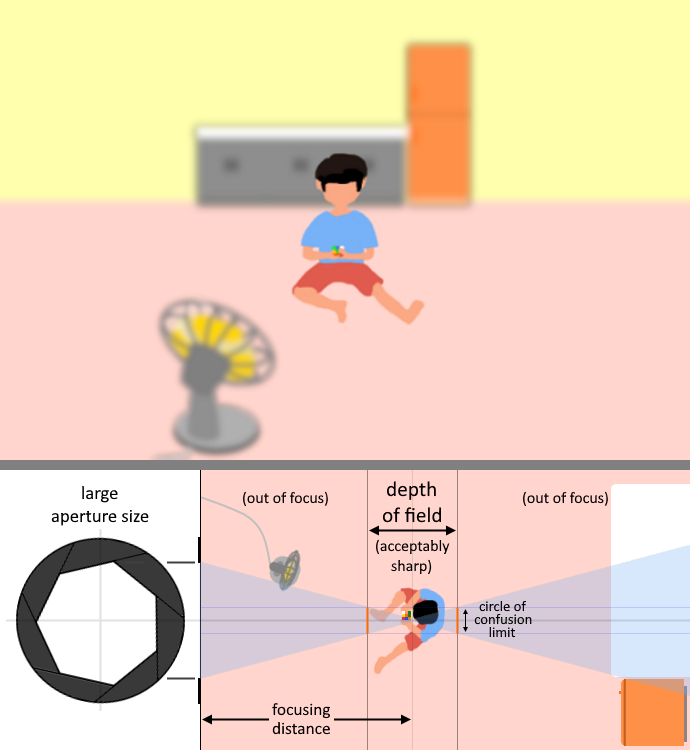With the same large aperture opening, we can also change our camera's focusing distance towards our foreground object (in this case, the electric fan) and make the rest of the scene blurry, as shown in the image below: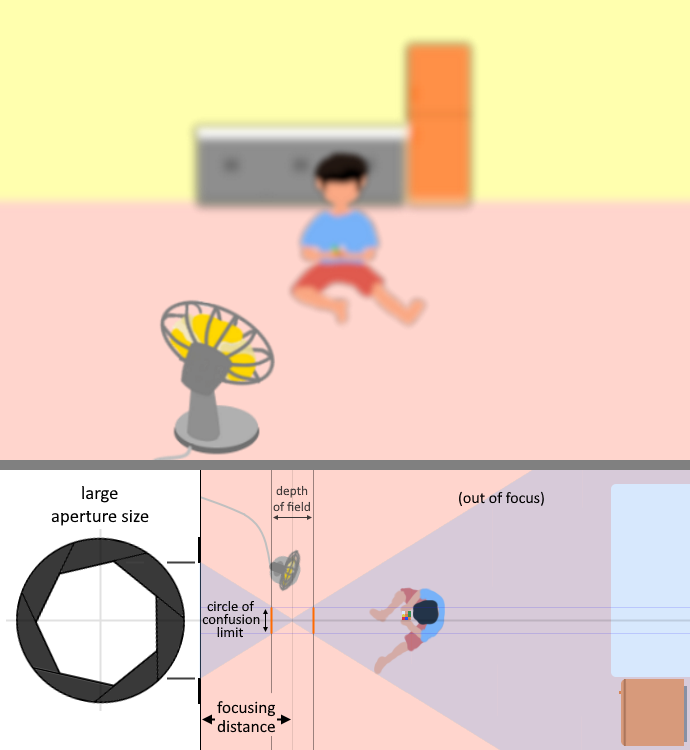By having a short focusing distance towards our subject and using a large aperture opening, we can see that the light spreads faster, resulting in a very narrow depth of field. The same thing happens when we take macro or close-up photos.

#### Focal length

As a rule of thumb, we use longer lenses when we want to take shallow depths of field shots. On the other hand, wide-angle lenses and lenses with short focal lengths are great for deep depth of field photography.

💡 If we want to focus on our subject and blur its surroundings, e.g., when taking portrait shots, we need a shallow depth of field. If, instead, we want to capture more objects in our scene, like when capturing an entire landscape view or a massive group photo, a deep depth of field is preferable.

In the next section of this text, we'll discuss how to use this DoF calculator. Then, we'll dive deeper into calculating the depth of field manually.

## How to use the DoF calculator?

Here are the steps you can follow when using our DoF calculator:

1. Select your camera's sensor size from the list. You can enter custom sensor width and height measurements by selecting Custom sensor size from the options.
2. Enter the focal length of the lens you are using.
3. Pick the aperture size you prefer to use.
4. Enter your approximate focusing distance to your subject.

Upon doing these steps, you'll get the depth of field and depth of field limits for your camera's settings. If you think the calculated depth of field is either too narrow or too wide for your liking, you can adjust your camera settings to meet your preference.

Our DoF uses a default value of 0.029 mm for the circle of confusion limit of a 35mm full-frame sensor size. You can click on the Advanced mode button below our calculator to change this value or modify the values of the factors that affect the circle of confusion limit. In the advanced mode, our DoF calculator will also display the corresponding focal ratio of your selected aperture f-stop, the approximate hyperfocal distance, and the hyperfocal near limit of your entered settings. We'll get more into these parameters in the next section of this text.

## How to calculate the depth of field?

We have two depth of field calculation formulas that we can use. In the previous section of this text, we mentioned that the depth of field is the distance between the depth of field far limit and the depth of field near limit. We can express that in an equation form like this:

$\small{DoF = DoF_{\text{far limit}}\ - DoF_{\text{near limit}}}$

That would be easy if we can right away physically measure the depth of field far and near limits. However, if we cannot measure them, we can calculate them using these formulas:

• $DoF_{\text{far limit}} = \frac{H\ \times\ u}{H\ -\ (u\ -\ f)}$

• $DoF_{\text{near limit}} = \frac{H\ \times\ u}{H\ +\ (u\ -\ f)}$

where:

• $H$ - Hyperfocal distance;
• $u$ - Focusing distance or the camera's distance to the subject;
• $f$ - Focal length of lens used.

Hyperfocal distance is the focusing distance in which we get the maximum depth of field, and we can calculate its value using this equation:

$\small{H = f + \frac{f^2}{N\ \times\ C}}$

where:

• $f$ - Focal length of lens used;
• $N$ - Aperture f-number; and
• $C$ - Circle of confusion limit.

The circle of confusion limit, which we know determines the depth of field, depends on several factors, as shown in the equation below:

$\small{C = \frac{\left(\frac{d_{\text{av}}}{d_{\text{sv}}\ \times \text{visual\ acuity}}\right)}{\text{enlargement}}}$

where:

• $d_{\text{av}}$ - Actual viewing distance of a printed photo version of an image; and
• $d_{\text{sv}}$ - Standard viewing distance that a person can observe the said printed photo through a defined visual acuity;
• $\text{visual\ acuity}$ - Resolution at which a typical viewer can distinguish details in the printed photo at the standard viewing distance in terms of line pairs per mm (lp/mm); and
• $\text{enlargement}$ - Enlargement factor of the image produced on the film or camera sensor into the printed image.

Enlargement factor is essentially the ratio of the diagonal of the printed image ($\small{\text{diagonal}_\text{p}}$) and the diagonal of the camera's sensor ($\small{\text{diagonal}_\text{s}}$). Expressed in equation form:

$\small{\text{enlargement} = \frac{\text{diagonal}_{\text{p}}}{\text{diagonal}_{\text{s}}}\times1000}$

To calculate these diagonals, we can use the Pythagorean theorem, as shown in the equations below:

• $\text{diagonal}_{\text{p}} = \sqrt{w_{\text{p}}^2 + h_{\text{p}}^2}$

• $\text{diagonal}_{\text{s}} = \sqrt{w_{\text{s}}^2 + h_{\text{s}}^2}$

where:

• $w_{\text{p}}$ - Width of print;
• $h_{\text{p}}$ - Height of print;
• $w_{\text{s}}$ - Width of sensor; and
• $h_{\text{s}}$ - Height of sensor.

🙋 Although we provided the formulas needed to find the circle of confusion limit, we usually approximate its value around 0.01 mm to 0.20 mm. You can also keep in mind that we also get a smaller circle of confusion limit with a smaller sensor size, therefore a shallower depth of field. But we would also have to shorten our focal length to capture the same shot. That results in an overall effect of a deeper depth of field. You can learn more about the impact of using different sensor sizes in our crop factor calculator.

## Alternate depth of field formula

Alternatively, we can also use this simplified depth of field calculation formula:

$DoF = \frac{2\ \times\ u^2\times\ N\times\ C}{f^2}$

where:

• $u$ - Focusing distance or the camera's distance to the subject;
• $N$ - Aperture f-number;
• $C$ - Circle of confusion limit; and
• $f$ - Focal length of lens used.

🙋 Please note that this simplified depth of field formula has some limitations in giving accurate results. Nevertheless, you can still use this in approximating different depths of fields.

Understanding depth of field can help you take great pictures, whether of people, still objects, or landscapes. But remember that as we change our camera's settings, especially the aperture size, we may also need to adjust our camera's exposure and shutter speed settings. You can check our exposure calculator and our shutter speed calculator to learn more about these other settings. 📸

## FAQ

### What is the depth of field of a 50 mm lens?

Let's say our camera has a circle of confusion, c, of 0.029 mm, and we set our camera with an f-stop of f/4 (focal ratio, N, of 4) and focus at a distance, u, around 1,200 mm. We can approximate DoF using: DoF = u² × 2 × N × C / f² or by following these steps:

1. Square u: 1,200 × 1,200 = 1,440,000.
2. Multiply it by 2, N, and c: 1,440,000 × 2 × 4 × 0.029 = 334,080.
3. Divide that by the square of the focal length: 334,080 / 50² = 133.632 mm ≈ 0.13 m.

### What controls the depth of field?

The depth of field depends mainly on the camera's aperture size. The smaller it is, the deeper the depth of field gets. The focal length of the lens used also affects the depth of field. Using a long lens narrows the depth of field. Moving to a closer focusing distance to your subject results in a narrower or shallower depth of field.

### How is the depth of field related to aperture size?

The smaller the aperture is, the deeper the depth of field becomes. That is because light rays are only allowed to scatter in slight deviations due to the small aperture opening for the light to enter. Expanding the aperture opening allows the light rays getting into our camera to spread wider, resulting in more parts of the image blurring.

### How do I get a shallow depth of field?

To get a narrow or shallow depth of field, you can make one or more of these changes to your camera setup:

• Widen your aperture opening;
• Use a long focal length lens; or
• Move closer to your subject.

### Does shutter speed affect the depth of field?

No, shutter speed does not affect the depth of field. However, you may have to widen your aperture to let more light in when you increase your camera's shutter speed. Or, you may have to reduce your aperture opening when taking with a long shutter speed. In those cases, the changes in aperture size could affect the depth of field. But changes in shutter speed settings by itself does not affect the depth of field.

Jasmine J Mah and Kenneth Alambra
Sensor size
35mm full-frame
Lens focal length (f)
mm
Aperture f-stop
f/2.8
Focusing distance (u)
ft
Results
Depth of field (DoF)
ft
DoF far limit
ft
DoF near limit
ft
People also viewed…

### Age on other planets

This calculator helps you compute your age and upcoming birthdays on other planets. You can either input your *Date of Birth* or your current *Age* to have the results.

### Classroom spacing

The classroom spacing calculator analyzes how may students can fit in the classroom while respecting social distancing rules.

### Lost socks

Socks Loss Index estimates the chance of losing a sock in the laundry.

### Sunbathing

Do you always remember to put on sunscreen before going outside? Are you sure that you use enough? The Sunbathing Calculator ☀ will tell you when's the time to go back under an umbrella not to suffer from a sunburn!